GeeksforGeeks App
Open AppBrowser
Continue

# SQL Query to Convert Datetime to Epoch

In this article, we are going to learn a SQL Query to convert data in Datetime data type to data in Epoch datatype. So, Epoch data is basically a BIGINT number which defines the number of seconds elapsed between the specified DateTime and 1st January 1970 at 00:00:00. To execute these queries, we would need first to add data into a table in the “Datetime” datatype and then use the DATEDIFF() function to find the difference in seconds. Finally, we would use the CAST() function to get a BIGINT value.

#### DATEDIFF() :

This function in SQL returns the difference in time between two Datetime values that have been specified as parameters. The type of difference between the objects like years, minutes, hours, etc. also has to be specified.

#### CAST():

The CAST() function in SQL converts a value returned by an expression into a specified datatype.

Step 1: Creation of a SQL Database

We will first need to create a Database to work with tables and data in SQL. For that, the following query is used:

Query:

`CREATE DATABASE sample_db;`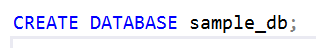Step 2: Specifying the usage of the created database

We need to start to use the created database. For that, the query that will be used is:

Query

`USE sample_db;`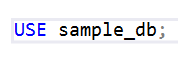Step 3: Creating a table with a DATETIME column

We need to create a table that has at least one column with a specified DATETIME datatype. We will use the following query :

Query:

```CREATE TABLE sample_table(valuesDatetime
DATETIME);```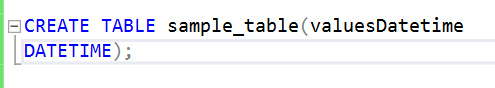Step 4: Inserting values into the Database

To convert “Datetime” values to Epoch values, we need to add them to the created table. For this operation, the following query will be used :

Query

```INSERT INTO sample_table(valuesDatetime)
VALUES
('20210115 08:15:32 AM'),
('20011012 01:23:11 PM'),
('20060524 07:16:45 PM');```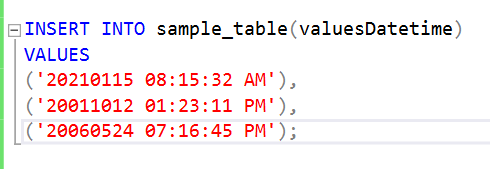Step 5: Getting epoch values corresponding to the Datetime values

We need to convert data from one data type to another, so first, we will select our data using the “SELECT command” in SQL. We will then pass the data that we have selected from our valuesDatetime column as a parameter to the DATEDIFF() function. The syntax of the DATEDIFF() function is :

```Syntax : DATEDIFF(part,start_datetime,end_datetime)
Parameters :
part : This is the unit in which the difference between the Datetime objects is returned.
start_datetime : Initial Datetime object
end_datetime : Final Datetime object
Returns : Difference between start_datetime and end_datetime in the unit defined in part parameter.```

To get epoch time, we will define our start_endtime as: ‘1970-01-01 00:00:00’ and our part parameter as s (to get epoch time in seconds). After this, we convert our result to BIGINT datatype using CAST()

Syntax:

` CAST(<expression> AS <datatype>)`

Query:

```SELECT CAST(DATEDIFF(s,
'1970-01-01 00:00:00',
valuesDatetime)
AS BIGINT)
FROM sample_table;```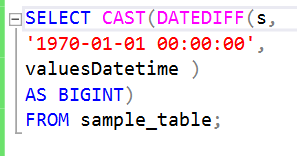Output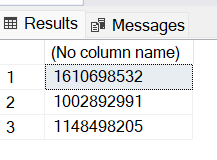My Personal Notes arrow_drop_up